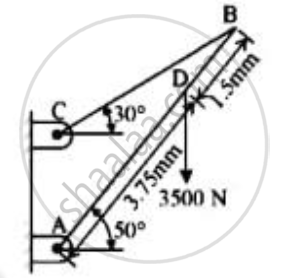# Determine the Tension in a Cable Bc Shown in Fig by Virtual Work Method. - Engineering Mechanics

Determine the tension in a cable BC shown in fig by virtual work method.Given: F=3500 N
ϴ = 50o
Length of rod = 3.75 mm + 1.5 mm = 5.25 mm
To find : Tension in cable BC

#### Solution

Let rod AB have a small virtual angular displacement θ in the clockwise direction

No virtual work will be done by the reaction force RA since it is not an active force
Assuming weight of rod to be negligible

Let A be the origin and dotted line through A be the X-axis of the system

 Active force(N) Co-ordinate of the point of action along the force Virtual displacement 3500 Y co-ordinate of D=yD=3.75sin θ 𝛿yD=3.75cos θ𝛿θ Tcos30 X co-ordinate of B=xB=5.25cos θ 𝛿xB=-5.25sin θ𝛿θ Tsin30 Y co-ordinate of B=yB=5.25sin θ 𝛿yB=5.25cos θ𝛿θ

By principle of virtual work :
-3500 x yd -Tsin 30 x yB -Tcos 30 x xB =0
-3500(3.75cos θ𝛿θ) – Tsin30(5.25cos θ𝛿θ) -Tcos30(-5.25sin θ 𝛿θ) = 0
Putting value of θ = 50o and dividing the above equation by 𝛿θ
(-3500 x 3.75cos50) – (Tsin30 x 5.25 cos50) + (Tcos30 x 5.25 sin50) = 0
5.25T(-sin30.cos50 + cos30.sin 50) = 3500 x 3.75 cos 50

T=(3500∗3.75c0s50)/(5.25(cos 30.sin50-sin30.cos50)
=(3500∗3.75cos50)/(5.25sin20)

=4698.4631 N
The tension in the cable BC is 4698.4631 N

Concept: Applications on Equilibrium Mechanisms
Is there an error in this question or solution?